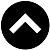-->

Type something and hit enter

# Magic formula of rule of 72. Why is it 72?

## Rule of 72

The rule of 72 is a simple formula to estimate the time that you need to double your investment based on your return.

For example, how long you need to double your investment given 20% return?

time = 72/x
time = 72/20
time = 3.6 years

How to proof?

1 x 1.2 x 1.2 x 1.2 x 1.2 = 2.07

Close enough right?

Ever wondered where did the 72 come from? Here’s the proof.
Given the formula for future value is

FV = PV x (1+x)^t

FV = Future Value
PV = Present Value
r = return
t = time

If we want to double our investment, our PV must be multiplied by 2, we end up with

2PV = PV (1+r)^t
2 = (1+r)^t

Let’s add natural logarithm to both sides

ln(2) = t ln(1+r)
t = ln(2) / ln(1+r)

According to taylor series expansion,

$ln\left(1+r\right)=x-\frac{{x}^{2}}{2}+\frac{{x}^{3}}{3}-\frac{{x}^{4}}{4}\dots ..$

However, if r is very small, we can safely assume that
Thus making

t = ln(2)/r
t = 0.693/r

Make it easier, we make it $×100$

t = 69.3/r

But hey! It is not 72 right?!

Yeah, yeah, let me explain. 69 is only easily divisible by 1,3,23,69.
To make this formula more convenient people decide to use 72 instead.

Note that 72 is divisible by 1,2,3,4,6,8,12,18,24,36,72.

To conclude, the rule of 72 is very good to estimate how long your investment can double. You might wonder, how far off is the value of using this formula?

Well, I have made a graph below, the higher the return is, the bigger the error

But this just serves as an estimation so it is good enough for most scenario.

Good enough right? You tell me

https://klse.i3investor.com/blogs/calculatingcandlestickwinrate/2020-09-14-story-h1513493062-Magic_formula_of_rule_of_72_Why_is_it_72.jsp